# Electronics and Communication Engineering - Electromagnetic Field Theory

36.

The torque (in mm) acting on a circular current loop of radius 1 mm in the xy plane, connected at the origin and with current 0.1 A, flowing in the sense of increasing in a magnetic field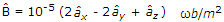is

 A.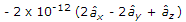B.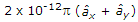C. 10-12p D. - 10-12p

Answer: Option B

Explanation:

t = M x B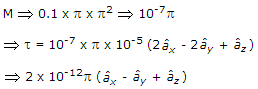37.

Which one of the following potential does not satisfy Laplace's equations?

 A. v = 10 xy B. v = p cos φ C.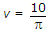D. v = f cos φ + 10

Answer: Option B

Explanation: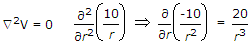.

38.

A rectangular waveguide measures 3 x 4.5 cm internally and has a 10 GHz signal propagated in it. Calculate the wave impedance for dominant mode.

 A. 130 p B. 1.3 p C. 120 p D. 12 p

Answer: Option B

Explanation:

Wave impedance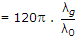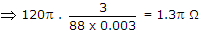.

39.

Calculate wave impedance for TE mode

 A. (377)Ω B. 226 Ω C. ∞ D. 629 Ω

Answer: Option D

Explanation: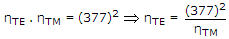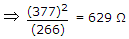.

40.

In a 100 turn coil, if the flux through each turn is (t3 - 2t)mWb, the magnitude of the induced emf in the coil at a time of 4 sec is

 A. 46 mV B. 56 mV C. 4.6 V D. 5.6 V

Answer: Option C

Explanation:

E = 100 (3t2 - 2)100(46)4600 mV4.6 V.

#### Current Affairs 2021

Interview Questions and Answers